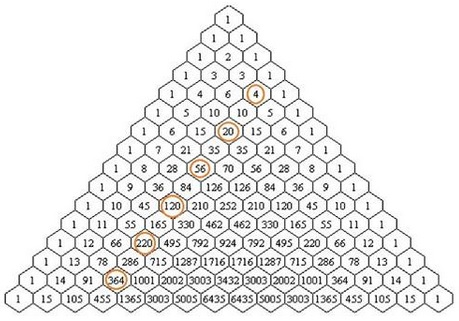# $\pi$ in Pascal's Triangle

Daniel Hardisky discovered $pi$ in Pascal's Triangle - something every one was looking for.

$\displaystyle\pi = 3+\frac{2}{3}\bigg(\frac{1}{C^{4}_{3}}-\frac{1}{C^{6}_{3}}+\frac{1}{C^{8}_{3}}-\cdot\bigg).$This is Daniel's modification of the famous Nilakantha Somayaji (1444-1544) series

\begin{align}\displaystyle \pi &=3+\frac{4}{2\cdot 3\cdot 4}-\frac{4}{4\cdot 5\cdot 6}+\frac{4}{6\cdot 7\cdot 8}-\ldots\\ &=3+\frac{4}{6}\bigg(\frac{1\cdot 2\cdot 3}{2\cdot 3\cdot 4}-\frac{1\cdot 2\cdot 3}{4\cdot 5\cdot 6}+\frac{1\cdot 2\cdot 3}{6\cdot 7\cdot 8}-\ldots\bigg)\\ &=3+\frac{2}{3}\bigg(\frac{1}{C^{4}_{3}}-\frac{1}{C^{6}_{3}}+\frac{1}{C^{8}_{3}}-\ldots\bigg) \end{align}

Daniel based his discovery on Tony Foster's observation that each of the denominators in Nilakantha's series is the area of a Pythagorean triangle. This is indeed so. All Pythagorean triples could be found from

$a=2mn,$ $b=m^{2}-n^{2},$ $c=m^{2}+n^{2},$

where $m$ and $n$ are integers. With so defined $a,b,c,$ it is easy to see that $a^{2}+b^{2}=c^{2}.$ The area of the corresponding triangle is, say, $A=ab/2=mn(m^{2}-n^{2}),$ which for $n=1$ gives $A=(m-1)m(m+1),$ such that the denominators in the series result for $m=5,7,9,\ldots$

Of course, the Leibniz series $\displaystyle\frac{\pi}{4}=\sum_{n=1}(-1)^{n+1}\frac{1}{2n-1}$ can also be looked at as the sum of the reciprocals of the binomial coefficients:

$\displaystyle\frac{\pi}{4}=1-\frac{1}{C^{3}_{1}}+\frac{1}{C^{5}_{1}}-\frac{1}{C^{7}_{1}}+\ldots$

In the same vein, another of Nilakantha's series, viz.,

$\displaystyle\frac{\pi}{8}=\frac{1}{2^{2}-1}+\frac{1}{6^{2}-1}+\frac{1}{10^{2}-1}+\ldots$

can be written in terms of the binomial coefficients:

\begin{align}\displaystyle \frac{\pi}{8}&=\frac{1}{2^{2}-1}+\frac{1}{6^{2}-1}+\frac{1}{10^{2}-1}+\ldots\\ &=\frac{2}{1\cdot 2\cdot 3}+\frac{6}{5\cdot 6\cdot 7}+\frac{10}{9\cdot 10\cdot 11}+\ldots\\ &=\frac{1}{6}\bigg(\frac{C^{2}_{1}}{C^{3}_{3}}+\frac{C^{6}_{1}}{C^{7}_{3}}+\frac{C^{10}_{1}}{C^{11}{3}}+\ldots\bigg) \end{align}

So that we have

$\displaystyle \pi=\frac{4}{3}\bigg(\frac{C^{2}_{1}}{C^{3}_{3}}+\frac{C^{6}_{1}}{C^{7}_{3}}+\frac{C^{10}_{1}}{C^{11}_{3}}+\ldots\bigg)$

but it is easy to be clever in a hindsight after the fact.

A different sort of presence of $\pi$ was discovered by Jonas Castillo Toloza in 2007; this one associated with the triangular numbers:

\begin{align}\displaystyle \pi-2&=\frac{1}{1}+\frac{1}{3}-\frac{1}{6}-\frac{1}{10}+\frac{1}{15}+\frac{1}{21}-\frac{1}{28}-\frac{1}{36}+\frac{1}{45}+\frac{1}{55}-\ldots\\ &=\frac{1}{C^{2}_{2}}+\frac{1}{C^{3}_{2}}-\frac{1}{C^{4}_{2}}-\frac{1}{C^{5}_{2}}+\frac{1}{C^{6}_{2}}-\frac{1}{C^{7}_{2}}-\frac{1}{C^{8}_{2}}-\frac{1}{C^{9}_{2}}+\frac{1}{C^{10}_{2}}+\frac{1}{C^{11}_{2}}-\ldots \end{align}

This is a remarkable series for $\pi$ that I could not find anywhere else. I'll give it two proofs: a short one that reduces it to Nilakantha's series, and another, due to Jonas Castillo Toloza, that thus gives an independent derivation of the latter. These deserve a dedicated page.### Pascal's Triangle and the Binomial Coefficients